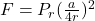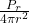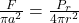## A microbe is ejected from its home planet by a violent event such as a volcanic eruption or a meteorite impact. The subsequent fate of the m

Question

A microbe is ejected from its home planet by a violent event such as a volcanic eruption or a meteorite impact. The subsequent fate of the microbe depends on the balance of the two main forces that act on it, namely, the gravitational attraction of the star around which the planet orbits and radiation pressure due to the luminosity of the star.

Required:
Model the microbe as a dark sphere of radius a at a distance r from the star. Assume that the total power radiated by the star P = 1.0 x 1031 J/s (greater than that of the sun by a factor of 30,000). Derive an expression for the radiation force on the microbe. Give your answer in terms of p, r,c and a.

in progress 0
6 months 2021-08-02T07:38:40+00:00 1 Answers 4 views 0Explanation:

The radiation pressure for a total absorption of the incident light is

P = S / c

Where S is the pointing vector, which is defined as the energy flux per unit area

S = I = P_r / A

where P_r  powre irradiate by star, A is the area over which the energy emitted by the star is distributed, which is the surface of a sphere

A = 4π r²

substituting

S =Pressure is defined by

P = F / A₂

Where A₂ is the area over which the force is applied, in this case the area of ​​the microbe

A₂ = π a²

P = F / π a²

we substitute